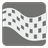Can You Pass?
Moderate+
English DE ES RU

If you have solved the "How to find friends" mission, then you already know how to check for the existence of a path in graphs. Let's try to add something more to that problem.

You are given a matrix (2D array) and the coordinates (row and column) of two cells with the same value. The matrix consists of digits. You may move to neighbouring cells either horizontally or vertically provided the values of the origin and destination cells are equal. You should determine if a path exists between two given cells.

A matrix is represented as a tuple of tuples with digits. Coordinates are represented as a tuple with two numbers: row and column. The result should be any value which can be converted into a boolean. If a path exists, then return True. Return False if there is none.Input: Three arguments. A matrix as a tuple of tuples with integers, first and second cell coordinates as tuples of two integers.

Output: The existence of a path between two given cells as a boolean or a value that can be converted to boolean.

Example:
```can_pass(((0, 0, 0, 0, 0, 0),
(0, 2, 2, 2, 3, 2),
(0, 2, 0, 0, 0, 2),
(0, 2, 0, 2, 0, 2),
(0, 2, 2, 2, 0, 2),
(0, 0, 0, 0, 0, 2),
(2, 2, 2, 2, 2, 2),),
(3, 2), (0, 5)) == True, 'First example'
can_pass(((0, 0, 0, 0, 0, 0),
(0, 2, 2, 2, 3, 2),
(0, 2, 0, 0, 0, 2),
(0, 2, 0, 2, 0, 2),
(0, 2, 2, 2, 0, 2),
(0, 0, 0, 0, 0, 2),
(2, 2, 2, 2, 2, 2),),
(3, 3), (6, 0)) == False,
```

How it is used: Sometimes we don't need the full pathfinding algorithms implementation and can use simplified realisation of these algorithms. It can be an useful skill to find a simple ways.

Precondition:
1 < len(matrix) ≤ 10
all(1 < len(row) ≤ 10 for row in matrix)
all(all(0 ≤ x < 10 for x in row) for row in matrix)
matrix[first][first] == matrix[second][second]
first != second